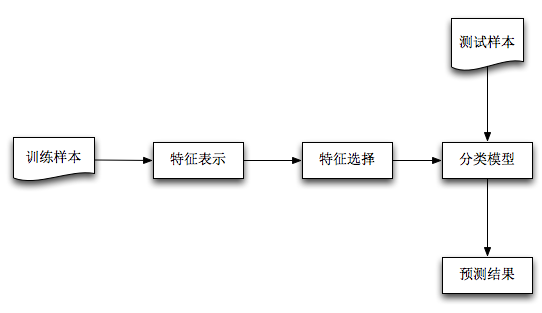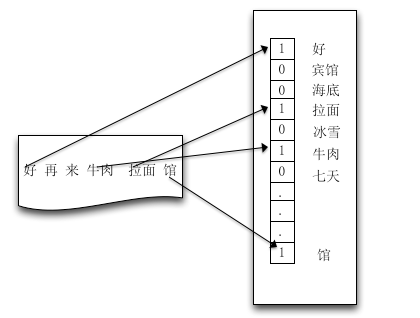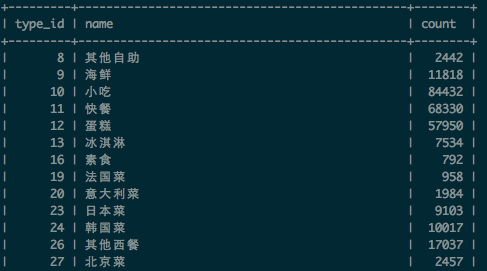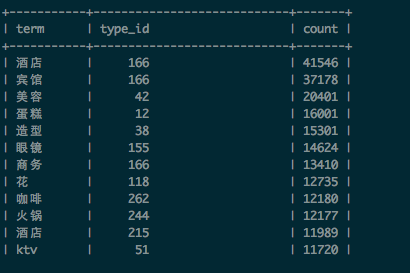前言特征表示A(i,j) = 特征词term(i) 在品类为type_id(j)出现的次数count

T(j) = 品类为type_id(j)在样本集出现的次数

N = 已校准POI数据集的数量

基于统计学习的特征选择算法

1.基于相关性度量(信息论相关) 2.特征空间表示(典型的如PCA)

$$[H(Y)=-\sum_{i=1}^{n}p\left ( y_{i}\right )\log p\left ( y_{i} \right )]$$

$$[H\left ( Y|X \right )=\sum _{x}p\left ( x \right )H\left ( Y|X=x \right )=-\sum _{x}\sum _{y}p\left ( x,y \right )\log p\left ( y|x \right )]$$

$$[IG(X)=H(Y)-H(Y|X)]$$

110火锅
011火锅
100小吃
101小吃

$$[H\left ( Y \right )=-\sum _{i=1}^{2}p\left ( y_{i} \right )\log p\left ( y_{i} \right )=-2*\frac{1}{2}*\log \frac{1}{2} = 1]$$$$[H\left ( C|A \right )=-\sum_{i=1}^{2}\sum_{j=1}^{2}p\left ( x,y \right )\log p\left ( y|x \right )=0-0- \frac{1}{2}\log \frac{2}{3} -\frac{1}{4}\log \frac{1}{3} =0.69]$$

分类模型

建模$$[p\left ( y|x \right )=\frac{p\left ( y \right )p\left ( x|y \right )}{p\left ( x \right )} \sim p\left ( x|y \right )]$$

多项伯努利模型

$$p(X|C_{j})=\prod_{i=1}^{|V|}=1(t_{i}\in X)p(t_{i}|C_{j}) %2B 1(t_{i}\notin X)(1-p(t_{i}|C_{j}))$$

多项事件模型

$$[p(X|C_{j})=p(w_{1},w_{2},w_{3}…w_{s}|C_{j})=\prod_{i=1}^{s}p(w_{i}|C_{j})]$$

$$[p(X|C_{j})=\prod_{i=1}^{|V|}p(t_{i}|C_{j})^{N(t_{i},X)}]$$

参数估计

$$[\Theta _{j}=p(y=C_{j})=\frac{\sum_{k=1}^{N}1(y_{k}=C_{j})}{\sum_{k=1}^{N}\sum_{j=1}^{C}1(y_{k}=C_{j})}=\frac{T\left ( j \right )}{N}]$$

$$[\Theta _{i|j}=p\left ( t_{i}|C_{j} \right )=\frac{\sum_{k=1}^{N}1(y_{k}=C_{j}1(t_{i}\in C_{j}))}{\sum_{k=1}^{N}1(y_{k}=C_{j})}=\frac{A(i,j)}{N}]$$

参数平滑小数溢出

$$[\log p(X|C_{j})=\log p(t_{1},t_{2},t_{3}…t_{|V|}|C_{j})=\sum_{i=1}^{|V|}\log p\left ( t_{i}|C_{j} \right )]$$

算法预测

• 对测试样本做中文分词，判断”牛肉“属于品类词，丢弃品类词”牛肉“前面的部分，并提取样本的特征词集合得到：[牛肉 拉面 馆]
• 根据字典，建立向量空间模型：x = [1, 0, 1, 1]
• 利用Naive Bayes模型分类预测，我们给出火锅和快餐两类样本的term_category_frequency统计：

21总结

• 机器学习解决问题最关键的一步是找准问题：这种问题能否用机器学习算法解决？是否存在其他更简单的方法？简单的如字符串匹配，利用正则就可以简单解决，才机器学习方法反而很麻烦，得不偿失。
• 如果能机器学习算法，如何去表示这个机器学习问题，如何抽取特征？又可能归类哪类机器模式（分类、聚类、回归？）
• 找准问题后，可以先尝试一些开源的机器学习工具，验证算法的有效性。如果有必要，自己实现一些机器算法，也可以借鉴一些开源机器学习算法实现。